心理学上有个术语叫做“第一印象效应”，大概是说人与人之间第一次直接或者间接交往中所接触到的信息，会在对方头脑中形成固有认识并占据主导地位。就如同提到美系SUV，大多数人脑海中浮现的第一印象是“厚重、宽大、舒适、大排量”，最后还一定忘不了加上一句“油老虎”。美系SUV都是油老虎？雪佛兰探界者表示这锅我们不背，并决定拉上福特翼虎来一场流言终结。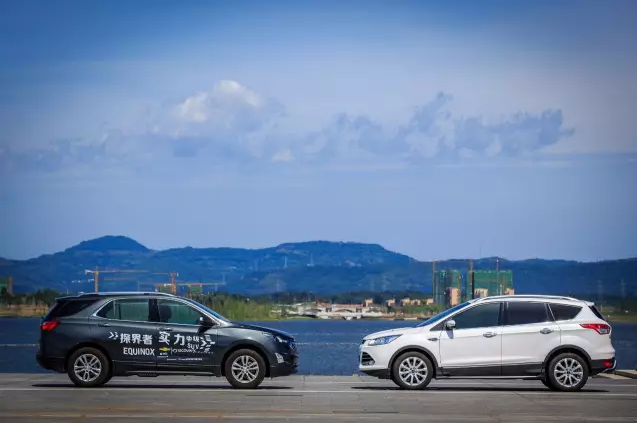放在二十年前乃至十年前，美系SUV好像还真没把油耗当一回事，可是哲学家又告诉我们世界上任何事物都是处在不断发展和变化中的，今天的美系SUV，以最新的代表车型雪佛兰探界者举个例子，已经在燃油经济性的优化上花了大量功夫，不光换用了“吃得少力不小”的小排量涡轮增压发动机，在整个车身的减重、降低风阻，乃至提升传动效率等方面都引入了诸多先进技术，那么美系SUV真的能甩掉“油老虎“的帽子吗？我们决定来一场真刀真枪的节油挑战赛。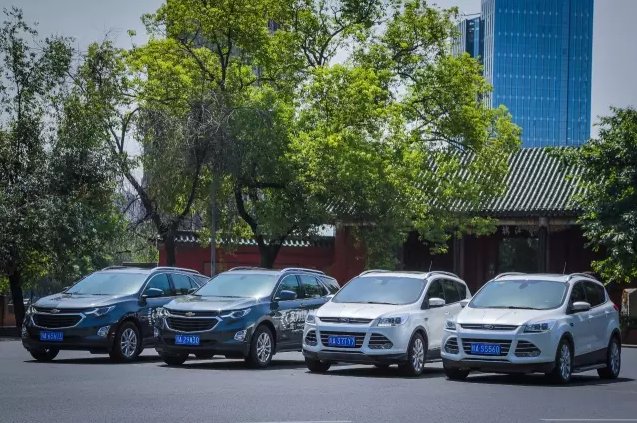我们是这样来测试的

■ 测试日期：2017年8月8日上午10点-下午4点

■ 天气状况：晴，气温31~36摄氏度

■ 测试车辆：4部测试车辆，分别是两部搭载1.5T涡轮增压发动机的雪佛兰探界者535T四驱拓界版、两部同样搭载1.5T涡轮增压发动机的长安福特翼虎四驱版。

■ 测试路线：起点选在成都市中心的核心商圈——太古里/IFS商圈，车队加满汽油从IFS出发，沿市区道路依次经由太古里、九眼桥、科华路和红星路南延线前往望江楼和环球中心，这是一段典型的城市工况路段，包括密集的交通灯、复杂多变的路况；之后的路线相对顺畅，从环球中心出发沿天府大道一路向南，经兴隆湖转入第二绕城高速，抵达三岔湖后，探界者和翼虎交换驾驶员后再返回到起点加油站，使用同一只加油枪再次补满燃油，加油量即为两车的燃油消耗量。整个测试路线往返里程共计约131公里，整个测试路线中城市拥堵路段、城市快速路和高速公路各占三分之一，而我们亦没有刻意省油地驾驶，因此更能体现车辆在综合路况下的与实际油耗表现。测试过程全纪录

上午10点我们准时从太古里出发，此时的成都市中心交通显然还没有从早高峰的余威下完全恢复，道路仍显得十分繁忙，而四处兴建的地铁工地无疑又对交通带来了额外的压力。在这样拥堵的路况下，相比已经上市多年的福特翼虎，探界者更具后发优势，凭借更新的轻量化车身结构和更多铝制材料的应用，探界者比同样搭载1.5T发动机和四驱系统的福特翼虎足足轻了一名成年男子的重量，加上35Nm的扭矩优势和最大扭矩到达时机更早，探界者在需要频繁起步加速的市区道路比翼虎多了一份从容，1/3的油门开度应付这样走走停停的路况便已足够；而反观翼虎，较弱的低扭和不算线性的输出往往让驾驶者掌握不好油门的开度，踩下很深的油门翼虎才不太情愿的加速，待到转速接近2000rpm涡轮介入，突如其来的扭力往往又需要驾驶者补上一脚刹车，让翼虎白白浪费了很多燃油。在环球中心短暂休整之后，车队进入到天府大道。天府大道更像是城市快速路与市区道路的结合，限速80km/h，但每隔数百米就有交通信号灯，且作为连结成都市高新区和天府新区的主干道，人车流量相当大，这一段车速相对较高且车速需要频繁变化的路段，实际上相当考验车辆的综合性能。探界者搭载的6速DSS智能启停变速箱面对不断变化的车速相当淡定，表现平顺且高效，同时智能四驱系统可根据路况主动切换为前轮驱动，比适时四驱模式下最多降低10%的油耗。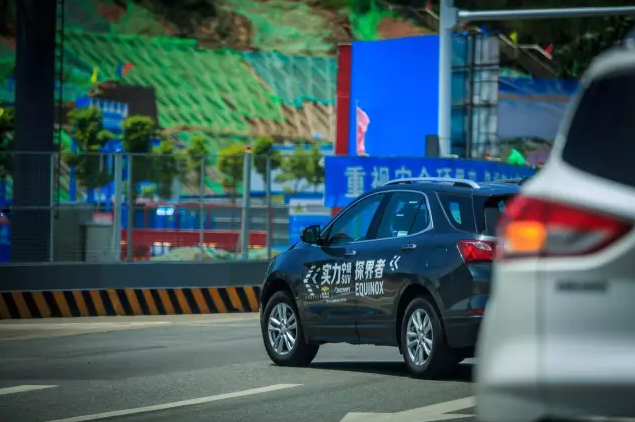第二绕城高速的路况就相当好了，几乎全高架的封闭道路铺装质量非常高，而且车流量很小，为了模拟真实驾驶环境，探界者和翼虎都按照法定最高限速行驶。在100km/h的巡航时速下，探界着和翼虎的发动机转速都保持在2000rpm上下，对于1.5T小排量涡轮增压发动机，这是一个兼顾燃油经济性和动力储备的经济转速，此时的表显瞬时油耗则都保持在5 L/100km之下。对于车身相对高大的SUV，高速行驶的噪音控制是个棘手的问题，探界者配备的ANC主动降噪功能这个时候就体现出了价值，即便以120km/h的时速巡航，车内乘员的交谈仍然非常轻松。数码界有句话叫做“买新不买旧“，在新技术迭代迅速的当下，把这句话用到汽车市场同样有道理。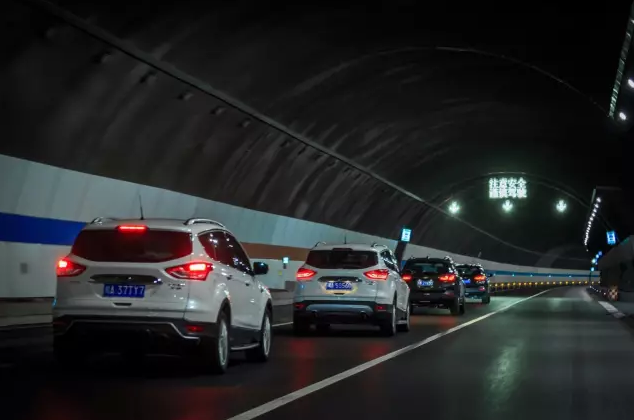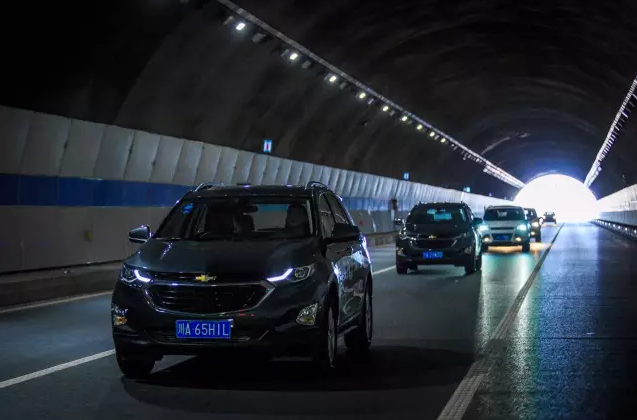第二绕城高速高速下来之后，经历一段窄小多弯的湖区道路，就到达了整个测试路段的折返点—三岔湖。探界者的尺寸要比翼虎更大，尤其是车身长度达到4652mm，比翼虎足足多出128mm，但得益于通用汽车最新一代的底盘结构以及通用汽车北美团队的悉心调教，多出的空间并未给探界者的灵活性带来负面影响，整部车在湖区道路上灵活轻巧，就连同行身材娇小的女司机对此也赞不绝口。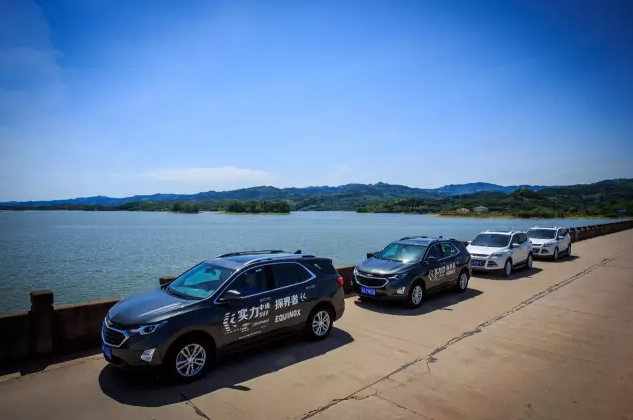好了，最后我们来看看4部车的油耗数据：

四驱版翼虎A行驶距离共计129.6公里，加油12.16L，综合百公里油耗9.38L；

四驱版翼虎B行驶距离共计132.0公里，加油13.72L，综合百公里油耗10.4L；

探界者A行驶距离共计132.1公里，加油量10.09L，综合百公里油耗7.64L；

探界者B行驶距离共计132.0公里，加油量9.91L，综合百公里油耗7.50L；

值得注意的是，探界者的表显油耗数字跟实际油耗数字基本吻合，而翼虎则要“乐观”了许多。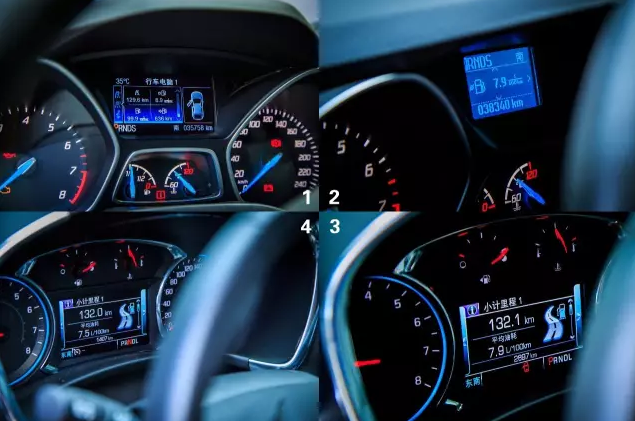上图仪表1234分别为翼虎A/翼虎B/探界者A/探界者B

小结：需要说明的是，参加测试的两台翼虎车龄稍高、车况一般，冷气也不够给力，测试当天空调几乎全负荷运转，所以如果是车况良好的翼虎新车，油耗数字应该会稍低一些，但无论如何，接近10L/100km的实际综合油耗对于一部紧凑型SUV已经是完全在合理范围之内。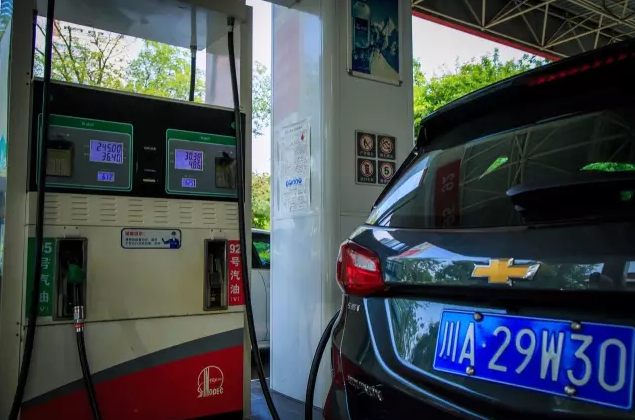而两台尺寸已经接近中型SUV的探界者，都取得了7.XL/100km的实测综合油耗，坦白说，这个结果甚至我们每一个人的意料。但通用汽车对新一代探界者“锱铢必较“的减重态度使其相比上一代车型足足轻了180Kg，加上1.5T小排量高效涡轮增压发动机和新一代6速手自一体DSS智能启停变速箱的配合，探界者能取得这样优异的油耗水平又是在情理之中。毫无疑问，探界者的出色表现已经证明：“美系SUV=油老虎“已经是过去式。##### 相关资讯

|分享

•已阅！握爪
•我手滑为你点赞
•128个赞！
•不明觉厉
•阅后既醉
•有钱！任性
•照片太美，我不敢看
•干货！杠杠的
•高大上
•膜拜中！
•请接受我的膝盖
•猫哥/猫妹么么哒
•神吐槽
•我只笑笑不说话
•我想静静
•窒息
##### 资讯相关车系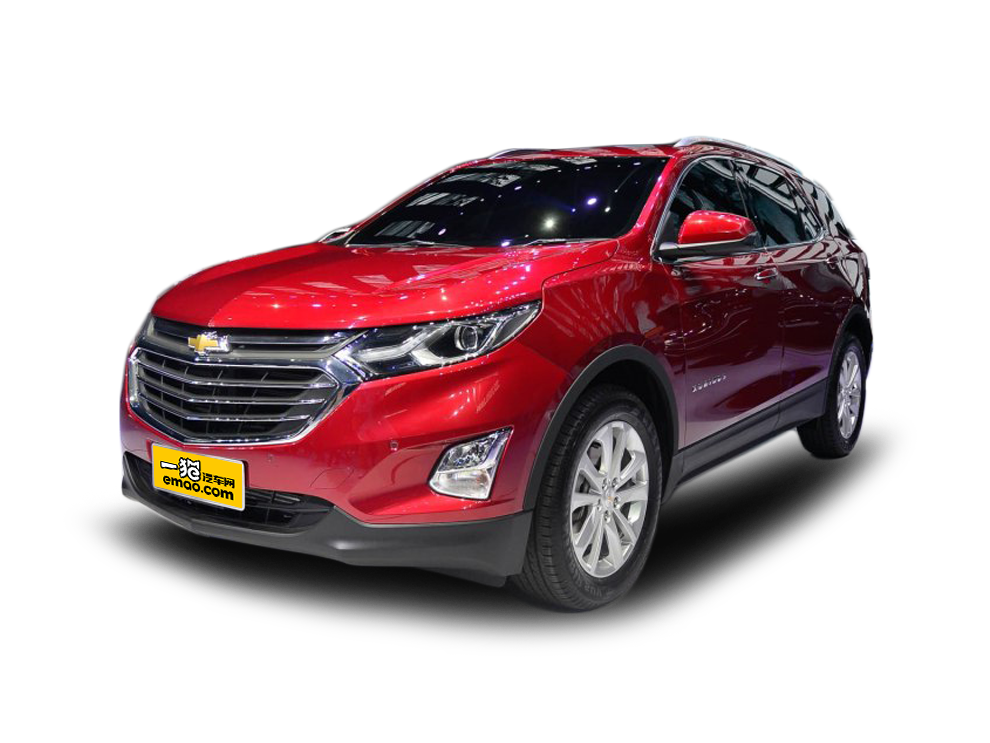##### 热度排行• 阿斯顿·马丁
• 奥迪
• 阿尔法罗密欧
• ALPINA

• 巴博斯
• 宝骏
• 宝马
• 保时捷
• 北汽制造
• 奔驰
• 奔腾
• 本田
• 比亚迪
• 标致
• 别克
• 宾利
• 布加迪
• 北汽威旺
• 北京
• 北汽绅宝
• 北汽幻速
• 北汽新能源
• 宝沃
• 比速汽车
• 北汽道达

• 昌河
• 长安
• 长城
• 长安商用
• 成功汽车
• 长江EV
• 长安轻车型

• 大众
• 道奇
• 东风
• 东风风神
• 东风小康
• 东南
• DS
• 东风风行
• 东风风度
• 东风风光

• 法拉利
• 菲亚特
• 丰田
• 福迪
• 福特
• 福田汽车
• 福汽启腾
• 风诺

• GMC
• 广汽传祺
• 广汽吉奥
• 观致

• 哈飞
• 海格
• 海马
• 华泰
• 黄海
• 恒天
• 红旗
• 哈弗
• 华颂
• 华凯
• 华泰新能源
• 汉腾汽车

• Jeep
• 江淮
• 江铃
• 捷豹
• 金杯
• 九龙
• 吉利汽车
• 金旅
• 金龙
• 江铃集团轻汽
• 江铃集团新能源
• 君马汽车
• 捷途

• 开瑞
• 凯迪拉克
• 科尼赛克
• 克莱斯勒
• KTM
• 卡威
• 凯翼
• 康迪
• 康迪电动汽车集团

• 猎豹汽车
• 兰博基尼
• 劳斯莱斯
• 雷克萨斯
• 雷诺
• 理念
• 力帆
• 莲花汽车
• 林肯
• 铃木
• 陆风
• 路虎
• 路特斯
• 领志
• 领克
• 零跑汽车

• MG
• MINI
• 马自达
• 玛莎拉蒂
• 迈凯伦
• 摩根

• 纳智捷

• 讴歌
• 欧宝
• 欧朗
• 欧拉
• 欧尚汽车

• 帕加尼

• 奇瑞
• 启辰
• 起亚
• 前途
• 庆铃汽车

• 日产
• 荣威
• 瑞麒汽车
• 如虎
• 瑞驰

• 上汽大通
• smart
• 三菱
• 双环
• 双龙
• 斯巴鲁
• 斯柯达
• 萨博
• 思铭
• 赛麟
• SWM斯威汽车

• TESLA
• 泰卡特
• 腾势

• 威麟
• 威兹曼
• 沃尔沃
• 五菱汽车
• 五十铃
• 潍柴英致
• WEY
• 蔚来
• 威马汽车

• 现代
• 雪佛兰
• 雪铁龙
• 西雅特
• 新特汽车
• 小鹏汽车
• 新宝骏

• 野马汽车
• 一汽
• 依维柯
• 英菲尼迪
• 永源
• 驭胜

• 中华
• 中兴
• 众泰
• 知豆
• 之诺
• 正道汽车
• A
• B
• C
• D
• E
• F
• G
• H
• I
• J
• K
• L
• M
• N
• O
• P
• Q
• R
• S
• T
• U
• V
• W
• X
• Y
• Z

• 阿斯顿·马丁
• 奥迪
• 阿尔法罗密欧
• ALPINA

• 巴博斯
• 宝骏
• 宝马
• 保时捷
• 北汽制造
• 奔驰
• 奔腾
• 本田
• 比亚迪
• 标致
• 别克
• 宾利
• 布加迪
• 北汽威旺
• 北京
• 北汽绅宝
• 北汽幻速
• 北汽新能源
• 宝沃
• 比速汽车
• 北汽道达

• 昌河
• 长安
• 长城
• 长安商用
• 成功汽车
• 长江EV
• 长安轻车型

• 大众
• 道奇
• 东风
• 东风风神
• 东风小康
• 东南
• DS
• 东风风行
• 东风风度
• 东风风光

• 法拉利
• 菲亚特
• 丰田
• 福迪
• 福特
• 福田汽车
• 福汽启腾
• 风诺

• GMC
• 广汽传祺
• 广汽吉奥
• 观致

• 哈飞
• 海格
• 海马
• 华泰
• 黄海
• 恒天
• 红旗
• 哈弗
• 华颂
• 华凯
• 华泰新能源
• 汉腾汽车

• Jeep
• 江淮
• 江铃
• 捷豹
• 金杯
• 九龙
• 吉利汽车
• 金旅
• 金龙
• 江铃集团轻汽
• 江铃集团新能源
• 君马汽车
• 捷途

• 开瑞
• 凯迪拉克
• 科尼赛克
• 克莱斯勒
• KTM
• 卡威
• 凯翼
• 康迪
• 康迪电动汽车集团

• 猎豹汽车
• 兰博基尼
• 劳斯莱斯
• 雷克萨斯
• 雷诺
• 理念
• 力帆
• 莲花汽车
• 林肯
• 铃木
• 陆风
• 路虎
• 路特斯
• 领志
• 领克
• 零跑汽车

• MG
• MINI
• 马自达
• 玛莎拉蒂
• 迈凯伦
• 摩根

• 纳智捷

• 讴歌
• 欧宝
• 欧朗
• 欧拉
• 欧尚汽车

• 帕加尼

• 奇瑞
• 启辰
• 起亚
• 前途
• 庆铃汽车

• 日产
• 荣威
• 瑞麒汽车
• 如虎
• 瑞驰

• 上汽大通
• smart
• 三菱
• 双环
• 双龙
• 斯巴鲁
• 斯柯达
• 萨博
• 思铭
• 赛麟
• SWM斯威汽车

• TESLA
• 泰卡特
• 腾势

• 威麟
• 威兹曼
• 沃尔沃
• 五菱汽车
• 五十铃
• 潍柴英致
• WEY
• 蔚来
• 威马汽车

• 现代
• 雪佛兰
• 雪铁龙
• 西雅特
• 新特汽车
• 小鹏汽车
• 新宝骏

• 野马汽车
• 一汽
• 依维柯
• 英菲尼迪
• 永源
• 驭胜

• 中华
• 中兴
• 众泰
• 知豆
• 之诺
• 正道汽车
• A
• B
• C
• D
• E
• F
• G
• H
• I
• J
• K
• L
• M
• N
• O
• P
• Q
• R
• S
• T
• U
• V
• W
• X
• Y
• Z

• 阿斯顿·马丁
• 奥迪
• 阿尔法罗密欧
• ALPINA

• 巴博斯
• 宝骏
• 宝马
• 保时捷
• 北汽制造
• 奔驰
• 奔腾
• 本田
• 比亚迪
• 标致
• 别克
• 宾利
• 布加迪
• 北汽威旺
• 北京
• 北汽绅宝
• 北汽幻速
• 北汽新能源
• 宝沃
• 比速汽车
• 北汽道达

• 昌河
• 长安
• 长城
• 长安商用
• 成功汽车
• 长江EV
• 长安轻车型

• 大众
• 道奇
• 东风
• 东风风神
• 东风小康
• 东南
• DS
• 东风风行
• 东风风度
• 东风风光

• 法拉利
• 菲亚特
• 丰田
• 福迪
• 福特
• 福田汽车
• 福汽启腾
• 风诺

• GMC
• 广汽传祺
• 广汽吉奥
• 观致

• 哈飞
• 海格
• 海马
• 华泰
• 黄海
• 恒天
• 红旗
• 哈弗
• 华颂
• 华凯
• 华泰新能源
• 汉腾汽车

• Jeep
• 江淮
• 江铃
• 捷豹
• 金杯
• 九龙
• 吉利汽车
• 金旅
• 金龙
• 江铃集团轻汽
• 江铃集团新能源
• 君马汽车
• 捷途

• 开瑞
• 凯迪拉克
• 科尼赛克
• 克莱斯勒
• KTM
• 卡威
• 凯翼
• 康迪
• 康迪电动汽车集团

• 猎豹汽车
• 兰博基尼
• 劳斯莱斯
• 雷克萨斯
• 雷诺
• 理念
• 力帆
• 莲花汽车
• 林肯
• 铃木
• 陆风
• 路虎
• 路特斯
• 领志
• 领克
• 零跑汽车

• MG
• MINI
• 马自达
• 玛莎拉蒂
• 迈凯伦
• 摩根

• 纳智捷

• 讴歌
• 欧宝
• 欧朗
• 欧拉
• 欧尚汽车

• 帕加尼

• 奇瑞
• 启辰
• 起亚
• 前途
• 庆铃汽车

• 日产
• 荣威
• 瑞麒汽车
• 如虎
• 瑞驰

• 上汽大通
• smart
• 三菱
• 双环
• 双龙
• 斯巴鲁
• 斯柯达
• 萨博
• 思铭
• 赛麟
• SWM斯威汽车

• TESLA
• 泰卡特
• 腾势

• 威麟
• 威兹曼
• 沃尔沃
• 五菱汽车
• 五十铃
• 潍柴英致
• WEY
• 蔚来
• 威马汽车

• 现代
• 雪佛兰
• 雪铁龙
• 西雅特
• 新特汽车
• 小鹏汽车
• 新宝骏

• 野马汽车
• 一汽
• 依维柯
• 英菲尼迪
• 永源
• 驭胜

• 中华
• 中兴
• 众泰
• 知豆
• 之诺
• 正道汽车
• A
• B
• C
• D
• E
• F
• G
• H
• I
• J
• K
• L
• M
• N
• O
• P
• Q
• R
• S
• T
• U
• V
• W
• X
• Y
• Z